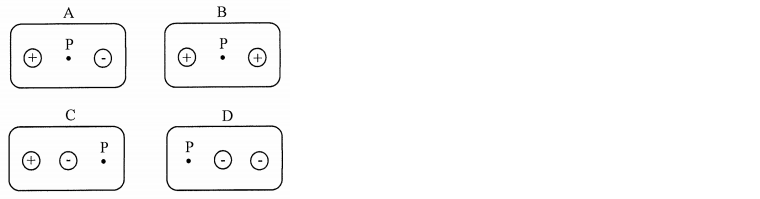Problem: In the four configurations of charges, all the charges are the same magniture, Q, but can be positive or negative. The distance between adjacent items is always the same. In which situation is the magnitude of the electric field at point P the largest? A) Situation A B) Situation B C) Situation C D) Situation D

82% (54 ratings)
Problem Details

In the four configurations of charges, all the charges are the same magniture, Q, but can be positive or negative. The distance between adjacent items is always the same. In which situation is the magnitude of the electric field at point P the largest?

A) Situation A

B) Situation B

C) Situation C

D) Situation D